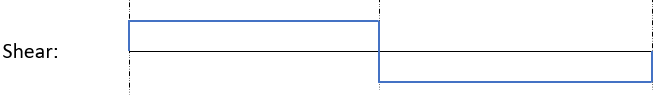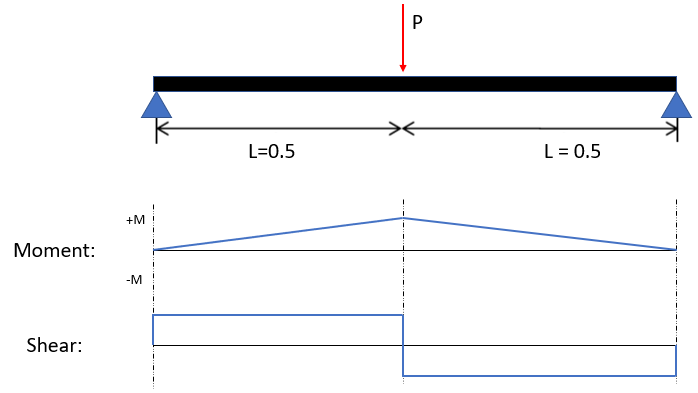## Shear & Moment Diagrams

Consider the below continuous beam with loading force P. Sketch an approximation of the shear and moment diagrams that best describe the scenario.Hint
Here is the shear diagram:The simply supported beam with only one force at the mid-span acting downwards will have reactions at the supports acting upwards. Therefore, the shear diagram will rise at the supports, be constant across until mid-span where the force is located. The shear diagram will drop vertically at mid span and then be constant again until the support at the end. Remember, shear is zero at the maximum moment in this example, which is at the middle point. The moment diagram is a diagonal line, since the shear diagram was vertical and horizontal lines.

(Note: If the beam had a distributed load across it, the shear diagram would have a diagonal line, and the moment diagram would be a parabola.)